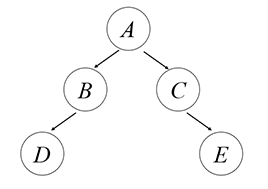1. ### 1807

输入一个字符串，求最长的包含且只包含1807这四个字符且按1→8→0→7顺序的子串的长度。

我一开始想的是$O(n^2)$的动态规划。后来发现不太对，就直接改成用一个字典来分别计算以1、8、0、7结尾的最长子串的长度，一次遍历$O(n)$

1. ### 【C、C++、C# 限定】牛牛的游戏俱乐部

$n$个游戏，每个游戏需要1单位时间来完成。第$i$个游戏必须在$t_i$时间或之前完成，完成则获得$w_i$分，没完成则扣除$w_i$分。求最大获分。

我的想法是用一个字典来储存每个时间段的所有游戏分数。然后每个时间段取最大的$t_i-t_{i-1}$个值减去所有剩下的值得出答案。然而语言成了我最大的障碍，特别是我平常用惯Python，各种变量类型声明整得我头大。

1. ### 平衡数

$[L,R]$区间内奇数位数字的和等于偶数位数字的和的数的个数。

由于上一题语言问题卡了许久，这题我就只写了个暴力解，通过13.33%。
数据范围是$10^9$，时间限制C、C++ 1秒，其它语言两秒。所以$O(n)$也基本会超时的。我暂时还想不出有啥更快的方法，希望大佬补充。

1. ### 中序遍历

字符串$A(B(C,),D(,E))$表示二叉树求这类字符串表示的二叉树的中序遍历。

结合用栈实现树的遍历。节点值按顺序入栈，空值也入栈。遇到逗号弹出两次，遇到右括号弹出一次。弹出顺序去掉空值即答案。

1. ### 是否凸多边形

给定$n$个点坐标，按顺序连成一个多边形，求该多边形是否凸多边形。

提示：对于一个多边形的任意边，若所有不在该边的点都在该边所在的直线的同一侧，则该多边形为凸多边形。

对于任意边的两点$(x_1,y_1)(x_2,y_2)$，用直线的两点式表示该边所在直线

$\frac{x-x_1}{x_1-x_2}=\frac{y-y_1}{y_1-y_2}$

对于其余点$(x_i,y_i)$分别代入该式的$x$$y$，若均为大于或小于，则都在同一侧，即该多边形为凸多边形。

16